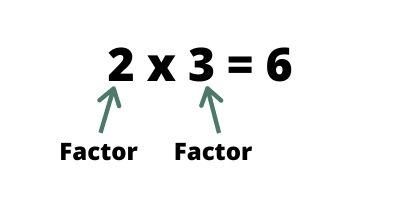# Factors of 36

## What is the factor?

Factors are integers you multiply together to get another integer. Also remember that the factors always include 1 and itself.NOTE: When finding the factors of a number, ask yourself “what numbers can be multiplied together to give me this number?.

## How to find the factors of 36?

THINK: What pairs of numbers can be multiplied together to give me 36?

Step 1: 1 x 36 = 36, so put these numbers in the factor list.

 1 … 36
Step 2: Take 2 and divide with 36. It gives 2 x 18 = 36.
 1 2 18 36
Step 3:Take 3 and divide with 36. It gives 3 x 12 = 36
 1 2 3 12 18 36
Step 4: Take 4 and divide with 36. It gives 4 x 9 = 36
 1 2 3 4 9 12 18 36

Step 5: Take 5 and divide with 36. The remainder will be 1. But factors always give 0 remainder. This means 5 is NOT a factor of 36.

Step 6: Take 6 and divide with 36. It gives 6 x 6 = 36

 1 2 3 4 6 9 12 18 36

Step 7: Take 7 and divide with 36. The remainder will be 1. But factors always give 0 remainder. This means 7 is NOT a factor of 36.

Step 8: Take 8 and divide with 36. The remainder will be 4. But factors always give 0 remainder. This means 8 is NOT a factor of 36.

Step 9: Take 9 and divide with 36. It gives 9 x 4 = 36

 1 2 3 4 6 9 12 18 36

Step 10: Take 10 and divide with 36. The remainder will be 6. But factors always give 0 remainder. This means 10 is NOT a factor of 36.

So the factors of 36 are 1, 2, 3, 4, 6, 9, 12, 18, 36

TIPS: Try to keep tables (at least till 20) on your finger tips. Revise tables every day## Is 36 a prime or composite number?

36 is a composite number not prime number because it has more than 2 factors.

What is composite number? – The composite numbers are the positive integers which have more than two factors.

What is prime number? – An integer number that is divisible by two distinct integers, 1 and itself.

## What is the prime factorization of 36

The prime factorization of 36 are  2 x 2 x 3 x 3 (i.e. 22 × 32)

## Factor pairs of 36

Factors are often given as pairs of numbers, which multiply together to give the original number. These are called factor pairs

What is factor pairs: Factor pairs are combinations of two factors that multiply together to give the original number.

Factor pairs of 36 are:
1 x 36 = 36
2 x 18 = 36
3 x 12 = 36
4 x 9 = 36
6 x 6 = 36
9 x 4 = 36
12 x 3 = 36
18 x 2 = 36
36 x 1 = 36

## Is 36 a square number?

Yes, 36 is a square number.
The square root of 36 is 6.
The square of 36 is 1296.

Hope you learned to solve the factors of 36.

Factors of 11
Factors of 18
Factors of 24
Factors of 28
Factors of 30
Factors of 32
Factors of 36
Factors of 40
Factors of 42
Factors of 45
Factors of 48
Factors of 56
Factors of 72

Translate »
Scroll to Top